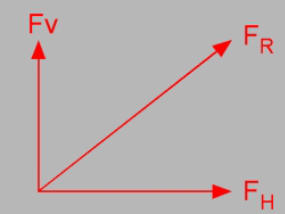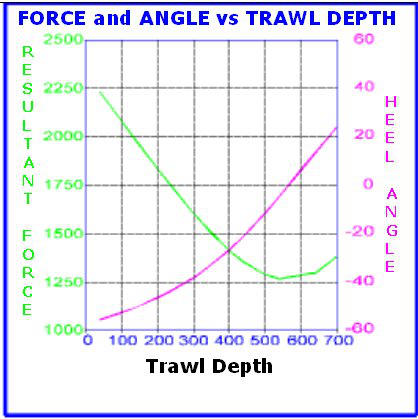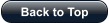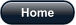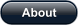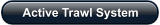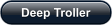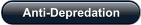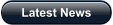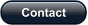Force Vectoring Principles Firstly, some very basic vector definitions: The term vector is used to indicate a quantity that has both magnitude and direction A vector is often represented by an arrow and a line segment. The length of the line represents the magnitude andthe arrow points in the direction of the vector A vector can be split into components e.g. at right angles to each other Vectors can be added together, subtracted and multiplied For a system to be in equilibrium, the vector sum must be equal to zero Now, applying these to trawling.... To position the trawl at a certain depth, the vertical component of the tension in the warp must be balanced by aforce acting in the opposite direction. This required force Fv is the vector sum of the weight component of the device and the vertical component of the lift generated by the device. The warp length and trawl speed are two variables which determine the required force. To spread the trawl, an equal and opposite horizontal component of force Fh is required from each device. The trawl speed and the fluid dynamic characteristics of the net determine the force required. (To position the trawl laterally, while maintaining the same spread, the horizontal component of the one device must be increased, while the horizontal component of the other device must be decreased by a similar amount.) The vector sum of the vertical force Fv and the horizontalforce Fh gives the resultant force vector FR. The length of this vector is equal to the magnitude of the liftforce that the device must generate, and the angle of FR tothe horizontal is equal to the heel angle of the device. This is illustrated graphically on the left. Thus, if the vertical and horizontal forces necessary toposition the trawl are known, the resultant force and heelangle can be derived. Below is a graph which typically illustrates the forces and heel angles required to maintain a trawl at a particulardepth. For example, for a depth of 500m the required resultant forces is 1300 kg and the heel angle -11 degrees. .